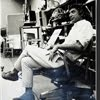## Eugene Ray SDSU 1970'sEugene Ray 1970's. photo credit: Tom Davis

## Wednesday, January 22, 2014

### 1970-1980 = RADIANT ARCHITECTURE / catalog and exhibitions

+ORGANIC BIOTRONICS+
>in our first decade of work in san diego<
+SDSU ENVIRON DESIGN+
>by 1980 it was visible in exhibitions<
>and we were winning competitions<
^^^^^^^^^^^^^^^^^^^^^^^^^^
>the great french architect, lecorbusier<
+GEOMANCY+
>developed in the orient forest<
^^^^^^^^^^^^^^^^^^^

>advanced the work of organic architecture<
>expressed by gaudi, wright, goff, soleri,
>etc.w/ a profound effort in biotronics<
^^^^^^^^^^^^^^^^^^^^^^^>the completion of the silver ship galvanized<
>my construction of a large exhibition and<
>i also made a catalog of the oeuvre<
^^^^^^^^^^^^^^^^^^^^^^^^
>twenty eight catalog plates<
>(a partial overview)<
^^^^^^^^^^^^^
>silver ship, prototype residence, iran library,
>california 21 shopping center<
^^^^^^^^^^^^^^^^^^^^^
seligman residence, wilty residence,
>jazz workshop, trailor apartment<
^^^^^^^^^^^^^^^^^^^^^^
>art & architecture building, restaurant<
^^^^^^^^^^^^^^^^^^^^^^^^^
>sdsu cafeteria interiors, project a & b<
^^^^^^^^^^^^^^^^^^^^^^^^^
>ferrocement residence, pictors restaurant,
>lajolla fabric shop, rail car conversion<
^^^^^^^^^^^^^^^^^^^^^^^^^^
>retrospective exhibitioon, museum of man<
>biotronic structure, biomorphic structure<
^^^^^^^^^^^^^^^^^^^^^^^^^
>new orleans prototypes, radiant yogurt shop
>pierucci plantation villa, escondido<
^^^^^^^^^^^^^^^^^^^^^^^^^

+BIOTRONICS 198O+
>as i have written explored affordable<
>prototypes based on systems that<
>could be easily assembled<
^^^^^^^^^^^^^^^^^^^
>kit of parts systems had been my focus<
>at LSU where two of my students<
^^^^^^^^^^^^^^^^^^^^^
>this success was continued at SDSU and<
>we won several competitions at large<
>universities throughout california<
^^^^^^^^^^^^^^^^^^^^^^^^
+BIOTRONICS 1990 EXHIBITION+
+WAS A VITAL REVELATION+
^^^^^^^^^^^^^^^^^^^^^^^^
eugene ray, mfa, architect
professor emeritus, sdsu